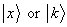# Physics 751: Midterm I Study Guide 2008

You should carefully read through all the notes I’ve posted on the web.

I will ask no questions on the historical stuff at the beginning, except for the purely physics content.   For example, you should have a good understanding of the Bohr atom, including the classical limit of a very large atom and how that fixes the quantum of angular momentum, and know how the Uncertainty Principle can be used to estimate the size of bound states, such as the atom on the table.

You should be sure you can do all the problems that were assigned for homeworks 1-4.

You should know Schrödinger’s equation by heart, the probability interpretation of the wavefunction, the conservation of probability current, and the expectation value of an operator.

Be able to find the time-dependent probability and current distribution for a superposition of states of different energies.

Know the definition of the delta function as a limit of a Gaussian wavepacket, and be able to Fourier transform a Gaussian wavepacket.  (Know the integral you need by heart.)   Also, know how to do Fourier series and transforms for simple functions, and be able to discuss the limitations of Fourier series for discontinuous functions.

You must know the bra and ket notation, be able to construct the first few elements of an orthogonal basis, the definitions of adjoint, Hermitian and unitary, and be able to diagonalize a  Hermitian or a unitary matrix.  Know the definition of determinant and trace, and how these relate to eigenvalues.

You should also feel comfortable going back and forth between the Schrödinger wavefunction notation and the Dirac bra ket notation.

Know the normalization conventions for the eigenkets of the momentum operator, and those of the position operator.  Know the identity in terms of these kets, and be able to use it to express a Schrödinger wave function as an integral overkets.  Be able to expressin terms of’s.

I expect you to be able to solve simple boundary-matching problems for square barriers, steps, square wells, and delta function potentials.